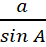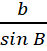### Trigonometry

Mathematics SSS 2 Third Term

WEEK 1

Trigonometry

Performance Objectives

Students should be able to;

1. Derive the sine rule
2. Apply the sine rule
3. Derive cosine rule
4. Apply cosine rule

Derivation of Sine Rule

Content

The sine rule formula states that the ratio of a side to the sine function applied to the corresponding angle is same for all sides of the triangle.

In any ΔABC, the angles are usually denoted by the capital letters A, B,C and the sides opposite these angles a, b,c respectively.

For a triangle ABC, sine rule can be stated as given below:

asin A= bsin BView More